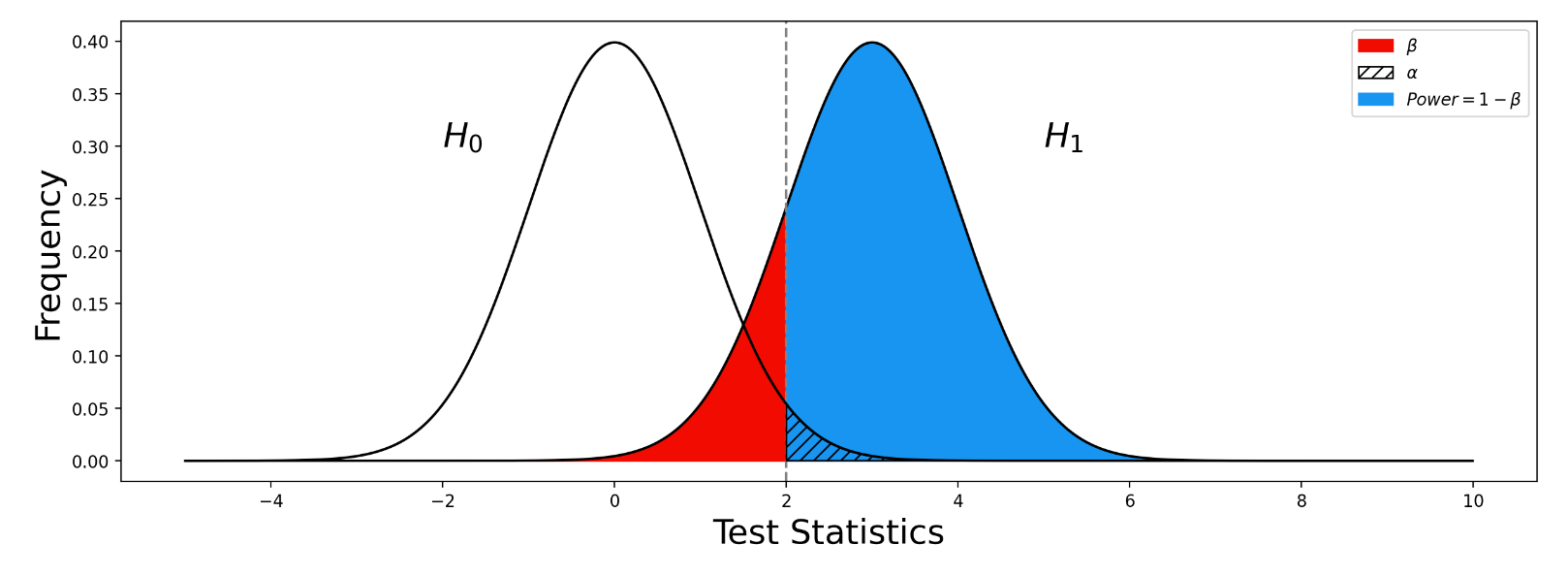# GWAS检验效能 Power analysis for GWAS

## 第一类错误，第二类错误以及检验效能

H0 为真 H0 为假$\alpha$ : 显著性水平$Power = Pr ( Reject\ | H_0\ is\ False) = 1 - \beta$## 影响检验效能的因素 Factors affecting power

• 总的样本量 Total sample size
• 病例与对照的比例 Case and control ratio
• 变异的效应量大小 Effect size of the variant
• 风险等位的频率 Risk allele frequency
• 显著性阈值 Significance threshold

## 非中心参数 Non-centrality parameter$y = \mu +\beta x + \epsilon$$\sigma^2 = Var(y) - Var(x)\beta^2$$\sigma^2 \thickapprox Var(y)$$Var(x) = 2f(1-f)$

•$f$ : 该变异的等位频率（allele frequency）$\lambda = ({{\beta}\over{SE_{\beta}}})^2$

## 数量表型的检验效能$\lambda = ({{\beta}\over{SE_{\beta}}})^2 \thickapprox N \times {{Var(x)\beta^2}\over{\sigma^2}} \thickapprox N \times {{2f(1-f) \beta^2 }\over {Var(y)}}$

•$CDF_{\chi^2}^{-1}(x)$ :$\chi^2$分布的累积分布函数的反函数$Power = Pr(\lambda > C ) = CDF_{\chi^2}(C, ncp = \lambda,df=1)$

•$CDF_{\chi^2}(x, ncp= \lambda)$ : 非中心参数NCP为$\lambda$$\chi^2$分布的累积分布函数

## 病例对照表型的检验效能 Power for large-scale case-control genome-wide association studies

•$P_{case}$ : 在病例中风险等位的频率 Risk allele frequency in cases
•$N_{case}$ : 病例的样本量 Number of cases. The total allele count for cases is then$2N_{case}$.
•$P_{control}$ : 在对照中风险等位的频率 Risk allele frequency in controls
•$N_{control}$ : 对照的样本量 Number of control. The total allele count for control is then$2N_{control}$.$z = {{P_{case} - P_{control}}\over {\sqrt{ {{P_{case}(1 - P_{case})}\over{2N_{case}}} + {{P_{control}(1 - P_{control})}\over{2N_{control}}} }}}$$Power = Pr(|Z|>C) = 1 - \Phi(-C-z) + \Phi(C-z)$

## 计算GWAS统计效能的网页工具 GAS power calculator

GAS power calculator工具实现了上述的计算方法，可以通过网页工具，指定参数后进行计算。

GAS power calculator

## 参考

• https://cloufield.github.io/GWASTutorial/20_power_analysis/
• Skol, A. D., Scott, L. J., Abecasis, G. R., & Boehnke, M. (2006). Joint analysis is more efficient than replication-based analysis for two-stage genome-wide association studies. Nature genetics, 38(2), 209-213.
• Johnson, J. L., & Abecasis, G. R. (2017). GAS Power Calculator: web-based power calculator for genetic association studies. BioRxiv, 164343.
• Sham, P. C., & Purcell, S. M. (2014). Statistical power and significance testing in large-scale genetic studies. Nature Reviews Genetics, 15(5), 335-346.